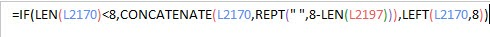# Useful Excel Formulaes## 1.To Trim the values.

LEFT(D8,LEN(D8)-3)

This will 1st calculate the length of the Char and then trim the last 3 characters.

## 2. To providing padding as space for cell.

What it does
1) Checks the length and validates – LEN(L2170)<8 font="">
2) If false (CONCATENATE(L2170,REPT(” “,8-LEN(L2197)))
* Here it concatenate the cell value with the space” “
* To find the number of time it check the  8 – length of the character.
* Which side it it needs to concatenate is decided by Left/Right

3. To find the duplicate entries with the n`th time it is repeating
“=IF(COUNTIF(A:A,A2)>1,COUNTIF(\$A\$1:A2,A2),0)

4. To find the difference between period Syntax:
DATEDIF (start_date, end_date, unit)

Example: `=DATEDIF(From;NOW();”D”)

DATEDIF= This is for the date diffference
From = From Period ( Do point to the Cell )
NOw= Current period or To Date
D / M / Y = Which denominates the period as date / Month / year